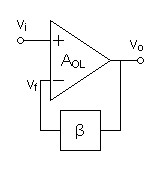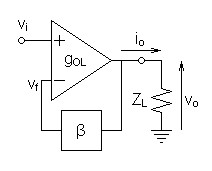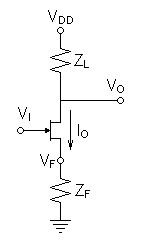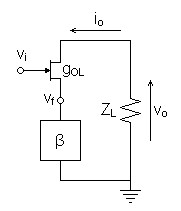Home  │ Audio Home Page

Updated September 26, 2022.  See Document History at end for details.

# Local Feedback

Is Local Feedback the same concept as Global Feedback?

### Introduction

The operation of a transistor with respect to the linearizing effect of a source or emitter resistor is so straightforward that it is often difficult to regard its operation as feedback in the same sense as global feedback.  Is local feedback the same mechanism as global feedback?  It the purpose of this article to examine this question by solving for the closed-loop feedback gain equations of circuits using global feedback and  local feedback.

### Feedback around a voltage gain block

The first circuit to examine applies global feedback to a differential input voltage gain block.  In all these circuits β represents a numerical value which when multiplied to the output value gives the voltage applied as feedback.  Usually, global feedback uses a voltage divider and local feedback uses an impedance to implement β, both usually resistive.

 Figure 1:  Circuit illustrating global feedback applied to a differential input voltage gain blockBeginning with the open loop gain equation, solve for the closed-loop feedback gain equation.

 (1) vo = AOL(vi - vf) (2) vo = AOL(vi - βvo) (3) vo = AOLvi - AOLβvo (4) vo + AOLβvo = AOLvi (5) vo(1 + AOLβ) = AOLvi

 (6) ACL = vo vi = AOL 1 + AOLβ

This result is the standard equation defining closed-loop gain for global feedback.  This is the reference to which the other equations are compared.  All feedback equations of this form (equations (6), (13), and (19)) reduce to
 (7) ACL ≈ 1 β
if AOLβ (or other equivalent term, such as gOLβ) is much greater than one.

### Feedback around a transconductance gain block

The second circuit to examine applies global feedback to a differential input transconductance gain block.  This is useful as an intermediate step because of the similarity to the transconductance gain of a transistor when feedback is applied.

 Figure 2:  Circuit illustrating global feedback applied to a differential input transconductance gain blockBeginning with the open loop gain equation, solve for the closed-loop feedback gain equation.  If β is implemented as a voltage divider, its contribution should be added to ZL.

 (8) vo = gOLZL(vi - vf) (9) vo = gOLZL(vi - βvo) (10) vo = gOLZLvi - gOLZLβvo (11) vo + gOLZLβvo = gOLZLvi (12) vo(1 + gOLZLβ) = gOLZLvi

 (13) ACL = vo vi = gOLZL 1 + gOLZLβ

This result is comparable to the to the standard equation above, except that the output impedance is in the equation.  Therefore loading can somewhat affect the gain result if the feedback factor is low.

 >

### Feedback around a transistor gain block

The last circuit to examine is a transistor amplifier with local feedback.  The circuit is meant to represent a generic transistor although it is illustrated as a JFET, and could represent a tube circuit as well.  The ac equivalent circuit makes the gate and source or base and emitter effectively differential inputs.  Because the output current also passes through the inverting input, the feedback impedance ZF by converting the output current to a feedback voltage at the feedback terminal becomes the feedback factor β.

 Figure 3:  Transistor amplifier with local feedback impedance. Figure 4:  AC equivalent circuit of same transistor amplifierBeginning with the open loop gain equation and substituting β for ZF, solve for the closed-loop feedback gain equation.

 (14) io = gOL(vi - vf) (15) io = gOL(vi - βio) (16) io = gOLvi - gOLβio (17) io + gOLβio = gOLvi (18) io(1 + gOLβ) = gOLvi

The resulting closed-loop transconductance gain equation is in the same mathematical form as to standard feedback gain equation of equation (6) above.
 (19) gOL = io vi = gOL 1 + gOLβ

where vo = ZLio, multiplying the closed-loop transconductance gain equation by ZL gives the closed-loop voltage gain.
 (20) AOL = vo vi = gOLZL 1 + gOLβ

dividing numerator and denominator by gOL and substituting back ZF for β gives the generic form of the most recognized common-emitter/common-source gain equation:
 (21) AOL = vo vi = ZL ZF + 1/gOL

Local feedback calculations take the same form as global ones and even return to the equation most associated with local feedback!

### Deductions

Having determined that the mechanism of local feedback is the same as that of global feedback, the differences attributed between them must pertain to differences in their open-loop gain blocks.  Individual transistors have comparatively low gain, a one-bend transfer curve, a very high frequency lowpass pole, minimal phase shift of about 90º, and a stable phase margin of about 90º.  Multiple transistor gain blocks have high gain, a more complicated transfer curve, and a lowpass pole greatly lowered by the necessity to compensate for 90º or more of phase shift per stage.  The following table summarizes these differences and others and their significance.

#### Gain Block Comparison

 Characteristic Multiple Transistor Gain Block Single Transistor Gain Block Winner Comments Open-loop Gain (AOL and gOL) high low multiple transistor Many of the multiple transistor gain block's faults are mitigated here by the high feedback factor (1 + AOLβ) that results from high open-loop gain. Transfer Curve1 possibly complicated one bend single transistor See first comment. Distortion low to vanishing high to low ? There may be virtue in multiple transistor gain block distortion when vanishingly low, however anything even slightly audible favors the euphony characteristic of a single transistor gain block.  Still a matter of debate. Output Impedance low to vanishing high to low multiple transistor? Low output impedance is usually a virtue, but not always. Lowpass Pole lower, compromised by phase compensation very high single transistor The multiple transistor gain block's disadvantage here may be mitigated by increasing gain bandwidth and possibly decreasing gain until the dominant pole is 10kHz or higher.2 Compensation Required yes no single transistor Maximum Phase Shift before Compensation ≈ number_of_stages × 90º ≈ 90º single transistor Compensated Phase Margin (180º - Phase Shift at unity open-loop gain) ≈ 45º ≈ 90º single transistor This factor relates to stability: 90º is associated with highest stability, 45º is associated with a small amount of ultrasonic overshoot and ringing on transients. Direct Coupling yes no multiple transistor

### Final Comment

I still hold out hope that operational amplifiers could gain virtue by attaining some charactistics of a single transistor, especially those of wide bandwidth and of a high frequency lowpass pole.

1See article Transfer Curve Shape and Distortion.
2
See article Musical Feedback Amplifiers.

Document History
August 27, 2011  Created.
September 26, 2022  Correct Compensated Phase Margin in chart where values transposed.